#### The planar case

For purposes of illustration, suppose that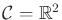. A configuration is expressed as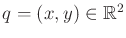, and a velocity is expressed as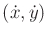. Eachis an element of the tangent space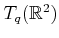, which is a two-dimensional vector space at every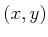. Think about the kinds of constraints that could be imposed. At each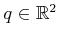, restricting the set of velocities yields some set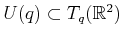. The parametric and implicit representations will be alternative ways to express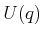for all.

Here are some interesting, simple constraints. Each yields a definition ofas the subset ofthat satisfies the constraints.

1.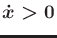: In this case, imagine that you are paddling a boat on a swift river that flows in the positivedirection. You can obtain any velocity you like in the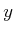direction, but you can never flow against the current. This means that all integral curves increase monotonically inover time.
2.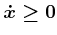: This constraint allows you to stop moving in thedirection. A velocity perpendicular to the current can be obtained (for example,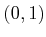causes motion with unit speed in the positivedirection).
3.,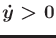: Under this constraint, integral curves must monotonically increase in bothand.
4.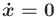: In the previous three examples, the set of allowable velocities remained two-dimensional. Under the constraint, the set of allowable velocities is only one-dimensional. All vectors of the form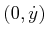for any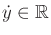are allowed. This means that no motion in thedirection is allowed. Starting at any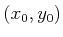, the integral curves will be of the form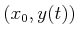for all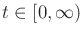, which confines each one to a vertical line.
5.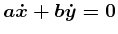: This constraint is qualitatively the same as the previous one. The difference is that now the motions can be restricted along any collection of parallel lines by choosing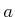and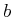. For example, if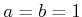, then only diagonal motions are allowed.
6.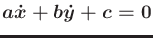: This constraint is similar to the previous one, however the behavior is quite different because the integral curves do not coincide. An entire half plane is reached. It also impossible to stop becasue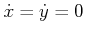violates the constraint.
7.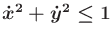: This constraint was used in Chapter 8. It has no effect on the existence of solutions to the feasible motion planning problem because motion in any direction is still allowed. The constraint only enforces a maximum speed.
8.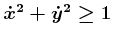: This constraint allows motions in any direction and at any speed greater than. It is impossible to stop or slow down below unit speed.
Many other constraints can be imagined, including some that define very complicated regions in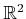for each. Ignoring the fact that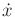and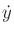represent derivatives, the geometric modeling concepts from Section 3.1 can even be used to define complicated constraints at each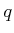. In fact, the constraints expressed above in terms ofandare simple examples of the semi-algebraic model, which was introduced in Section 3.1.2. Just replaceandfrom that section byandhere.

If at everythere exists some open set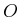such that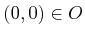and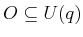, then there is no effect on the existence of solutions to the feasible motion planning problem. Velocities in all directions are still allowed. This holds true for velocity constraints on any smooth manifold .

So far, the velocities have been constrained in the same way at every, which means thatis the same for all. Constraints of this kind are of the form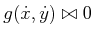, in which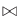could be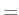,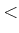,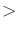,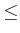, or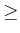, and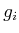is a function fromto. Typically, therelation drops the dimension of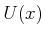by one, and the others usually leave it unchanged.

Now consider the constraint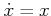. This results in a different one-dimensional set,, of allowable velocities at each. At each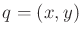, the set of allowable velocities must be of the form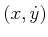for any. This means that asincreases, the velocity in thedirection must increase proportionally. Starting at any positivevalue, there is no way to travel to the-axis. However, starting on the-axis, the integral curves will always remain on it! Constraints of this kind can generally be expressed as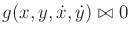, which allows the dependency onor.

Steven M LaValle 2020-08-14